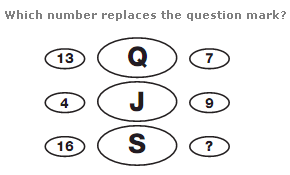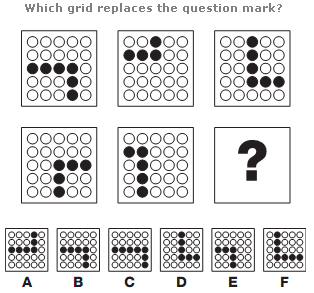# Puzzles - Logical puzzles

### Exercise :: Logical puzzlesAnswer : B Grid Explanation : Working in rows, left to right, top row then bottom, one black circle is added each time, with the positions of the circles moving (1/4) turn clockwise each time.

View Answer & ExplanationAnswer : 6 Explanation : In each row of the diagram, the numerical value of the middle letter equals the sum of the left and right hand numbers, subtracting 3.

View Answer & ExplanationAnswer : A Explanation : In each diagram, there are 2 lines of black dots, joining two sides together. The sides joined by the dots moves 1/4 turn clockwise as you move from left to right.

View Answer & Explanation

#### Current Affairs 2019

Interview Questions and Answers